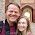## Wednesday, October 9, 2013

### Weak Instruments with a Small Panel

This post is in response to a comment by Luis Enrique on my previous post:

"As somebody who regularly consumes cross-country empirical research based on IV
regressions with samples of 50-100, I found this quite alarming. But then most
of the papers I read will be panel, with T of let's say 50.

This question may reveal shocking ignorance, but if the number of observations
in a panel (N*T) is say 100 * 50, does that translate into a (very) safe
sample size?" - Luis

My response:

Thanks for asking. I am no expert on time series so my opinion should really
be regarded with a grain of salt. First off, the key to remember is that the
problem is the result of a weak instrument. As the first stage R-squared on
the 2SLS approaches 1 the IV estimator becomes the OLS estimator which has
the same properties of OLS.

However, as the first stage R-squared approaches zero we start suffering
serious problems with IV in terms of both bias and efficiency.

Based on the cross sectional simulation, I would say that given a similarly
weak instrument, if each year's observation within each country is independent
then a panel of 100*50 should not be a problem. However, we expect explanatory
variables to be serially correlated and instruments to be often a 1 time only policy
changes which are extremely serially correlated which reduces the effective sample size
since each observation of the instrument can no longer be seen as independent.

Let's see how data generated in this manner may behave.

* First we define the original weakreg simulation in which there is
```* only cross sectional data.

cap program drop weakreg
program weakreg, rclass
clear
set obs `1'
* The first argument of the weakreg command is the number of
*  observations to draw.
gen z = rnormal()
gen w = rnormal()
gen x = z*.2 + rnormal() + w
gen u = rnormal()
gen y = x + w + u*5
reg y x
return scalar reg_x = _b[x]
return scalar reg_se_x = _se[x]
ivreg y (x=z)
return scalar ivreg_x = _b[x]
return scalar iv_se_x = _se[x]
end

* Now we define the original weakreg2 simulation in which there is
* panel data.

cap program drop weakreg2
program weakreg2, rclass
clear
set obs `1'
* The first argument of the weakreg command is the number of
*  clusters to draw.
gen id = _n

* There is a one time policy change
gen z = rnormal()

* The second argument is the number of observations in each cluster
expand `2'

bysort id: gen t = _n

gen w = rnormal()

* There is no policy effect before half way through the time period.
replace z = 0 if (t < `2'/2)

gen x = z*.2 + rnormal() + w

gen u = rnormal()

gen y = x + w + u*5 if t==1
bysort id: replace y = x + w + u*12.5^.5 + u[_n-1]*12.5^.5 if t>1

reg y x
return scalar reg_x = _b[x]
return scalar reg_se_x = _se[x]
ivreg y (x=z), cluster(id)
return scalar ivreg_x = _b[x]
return scalar iv_se_x = _se[x]
end

* Looking at first the cross sectional data:
simulate reg_x=r(reg_x)     reg_se_x=r(reg_se_x)  ///
ivreg_x=r(ivreg_x) iv_se_x=r(iv_se_x)    ///
, rep(1000): weakreg 5000
sum

/*
Variable |       Obs        Mean    Std. Dev.       Min        Max
-------------+--------------------------------------------------------
reg_x |      1000    1.490732    .0499934    1.29779   1.661524
reg_se_x |      1000    .0500304    .0007235   .0481176   .0520854
ivreg_x |      1000    .9938397    .3684834  -.2149411   1.970256
iv_se_x |      1000    .3638622     .037906   .2568952   .5094048

*/

* We see everything seems to be working very well.

* Looking now at the case where there is 50 clusters and 100 observations
* in each of them.

simulate reg_x=r(reg_x)     reg_se_x=r(reg_se_x)  ///
ivreg_x=r(ivreg_x) iv_se_x=r(iv_se_x)    ///
, rep(1000): weakreg2 50 100
sum

/*
Variable |       Obs        Mean    Std. Dev.       Min        Max
-------------+--------------------------------------------------------
reg_x |      1000    1.493905    .0508205   1.269596   1.670965
reg_se_x |      1000    .0502538    .0007578   .0478791   .0531899
ivreg_x |      1000    1.021512    .7299972  -1.083777    3.64094
iv_se_x |      1000    .7110581    .1806363   .3299663   1.566804
*/

* We can see that there is no huge bias in the ivregression though the
* standard errors are about twice that of the cross sectional data.

* Looking at first the cross sectional data:
simulate reg_x=r(reg_x)     reg_se_x=r(reg_se_x)  ///
ivreg_x=r(ivreg_x) iv_se_x=r(iv_se_x)    ///
, rep(1000): weakreg 1000
sum

/*
Variable |       Obs        Mean    Std. Dev.       Min        Max
-------------+--------------------------------------------------------
reg_x |      1000    1.483811    .1117863   1.130268     1.8281
reg_se_x |      1000    .1121205    .0034219   .1026788      .1249
ivreg_x |      1000    .9028068    .9626959  -8.379108   4.424785
iv_se_x |      1000    .9215948    .7681726   .4671113   23.61619
*/

* We can see the IV estimator has a large variance and a significant bias
* though it seems to be doing well in general.

simulate reg_x=r(reg_x)     reg_se_x=r(reg_se_x)  ///
ivreg_x=r(ivreg_x) iv_se_x=r(iv_se_x)    ///
, rep(1000): weakreg2 50 20
sum

/*
Variable |       Obs        Mean    Std. Dev.       Min        Max
-------------+--------------------------------------------------------
reg_x |      1000     1.49184    .1104733   1.148331   1.927401
reg_se_x |      1000    .1125144    .0040137   .1009569   .1271414
ivreg_x |      1000    .8209362    5.213284  -151.3602   17.03425
iv_se_x |      1000    10.00779    257.6449   .5320991   8149.177
*/

* We can see the IV estimator though dealing with the same number of
* observations has a huge variance and an even larger bias!
```
```* The take away is that even small panels seem like they work so long
* as there is sufficient observations over time.

Formatted By Econometrics by Simulation
```

1.1.